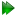Subsections

Torsionless pseudo-ellipse equations

Figure 16.19: The torsionless pseudo-ellipse model simulated and calculated in-frame Daeg(1) frame order matrix elements. In these plots, θX corresponds to the cone opening half-angle θx and θY to the cone opening half-angle θy. When the half-angle is not varied, the angle is fixed to either θx = π/4 or θy = 3π/8. Frame order matrix values have been calculated every 10 degrees. The first angle for the calculated elements is set to 0.01 degrees as a pseudo-ellipse cone opening angle of 0.0 cannot be correctly handled by the numerical integration.Figure 16.20: The torsionless pseudo-ellipse model simulated and calculated in-frame Daeg(2) frame order matrix elements. In these plots, θX corresponds to the cone opening half-angle θx and θY to the cone opening half-angle θy. When the half-angle is not varied, the angle is fixed to either θx = π/4 or θy = 3π/8. Frame order matrix values have been calculated every 10 degrees. The first angle for the calculated elements is set to 0.01 degrees as a pseudo-ellipse cone opening angle of 0.0 cannot be correctly handled by the numerical integration.Figure 16.21: The torsionless pseudo-ellipse model simulated and calculated out-of-frame Daeg(1) frame order matrix elements. In these plots, θX corresponds to the cone opening half-angle θx and θY to the cone opening half-angle θy. When the half-angle is not varied, the angle is fixed to either θx = π/4 or θy = 3π/8. Frame order matrix values have been calculated every 10 degrees. The first angle for the calculated elements is set to 0.01 degrees as a pseudo-ellipse cone opening angle of 0.0 cannot be correctly handled by the numerical integration.Figure 16.22: The torsionless pseudo-ellipse model simulated and calculated out-of-frame Daeg(2) frame order matrix elements. In these plots, θX corresponds to the cone opening half-angle θx and θY to the cone opening half-angle θy. When the half-angle is not varied, the angle is fixed to either θx = π/4 or θy = 3π/8. Frame order matrix values have been calculated every 10 degrees. The first angle for the calculated elements is set to 0.01 degrees as a pseudo-ellipse cone opening angle of 0.0 cannot be correctly handled by the numerical integration.Torsionless pseudo-ellipse rotation matrices

Setting the torsion angle σ to zero in the full torsion-tilt rotation matrix of equation 12.74c, the matrix becomes

 R(θ, φ) =. (16.55)

Torsionless pseudo-ellipse frame order matrix

The frame order matrix isThe surface normalisation factor isTorsionless pseudo-ellipse 1 degree frame order

The 1 degree frame order matrix with tensor rank-2 consists of the following elementsAs the trigonometric functions of θmax cannot be symbolically integrated, these components must be numerically integrated.

Torsionless pseudo-ellipse 2 degree frame order

The 2 degree frame order matrix with tensor rank-4 consists of the following elements, using Kronecker product double indices from 0 to 8Torsionless pseudo-ellipse frame order matrix simulation and calculation

The frame order matrix element simulation script from Section 16.2, pagewas used to compare the implementation of equations 16.58 and 16.59 above. Frame order matrix Daeg(1) and Daeg(2) values were both simulated and calculated, both within and out of the motional eigenframe. The in-frame Daeg(1) values are shown in figure 16.19 and Daeg(2) in figure 16.20. The out-of-frame Daeg(1) values are shown in figure 16.21 and Daeg(2) in figure 16.22.

The relax user manual (PDF), created 2020-08-26.